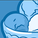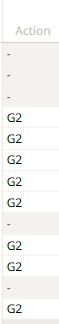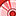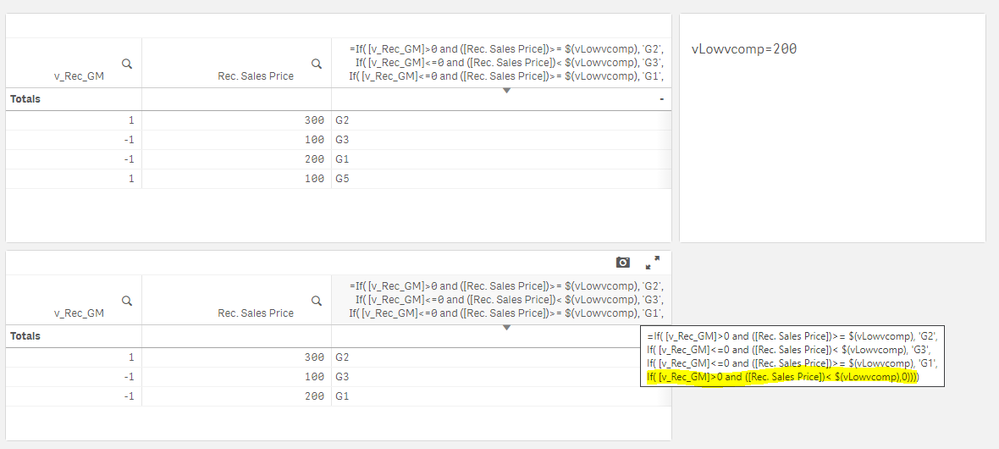# App Development

Discussion board where members can learn more about Qlik Sense App Development and Usage.

Announcements
April 22, 2PM EST: Learn about GeoOperations in Qlik Sense SaaS READ MORE
cancel
Showing results for
Search instead for
Did you mean:Creator

## Qlik Sense chart expression selection

HI,

I have added these two expressions in my Qlik Sense app.

Expression1>

If( \$(v_Rec_GM)>0 and ([Rec. Sales Price])>= \$(vLowvcomp), 'G2',
If( \$(v_Rec_GM)<=0 and ([Rec. Sales Price])< \$(vLowvcomp), 'G3',
If( \$(v_Rec_GM)<=0 and ([Rec. Sales Price])>= \$(vLovwcomp), 'G1',
If( \$(v_Rec_GM)>0 and ([Rec. Sales Price])< \$(vLowvcomp),'G5',null)))).

I am using this expression1 for the column = 'Action Item'

Expression2>

If( \$(v_Rec_GM)>0 and ([Rec. Sales Price])>= \$(vLowvcomp), 'G2',
If( \$(v_Rec_GM)<=0 and ([Rec. Sales Price])< \$(vLowvcomp), 'G3',
If( \$(v_Rec_GM)<=0 and ([Rec. Sales Price])>= \$(vLowvcomp), 'G1',
)))

I am using this expression2 for the column = 'Action'

Below are the columns that I obtain following the expressions-My Target->

I need to remove all the rows with 'G5' in my final app. and I will have 'Action Item' column with 'G1','G2', and 'G3'

only.

The issue I encountered is, removing 'G5' in the expression will fill up the column with '-' values, and I am not able to remove them in the chart.

Labels (1)
• ### Qlik Sense

9 RepliesSpecialist

Have you un-checked the "Include null values" box for the Action dimension in the chart properties panel?Creator
Author

@GaryGilesyes I have unchecked the boxCreator
Author

Now i have also tried the issue to resolve using below 'LEN' expression, but having same output with '-'

as values.

IF(len(If( \$(v_Rec_GM)>0 and ([Rec. Sales Price])>= \$(vLowcomp), 'G2',
If( \$(v_Rec_GM)<=0 and ([Rec. Sales Price])< \$(vLowcomp), 'G3',
If( \$(v_Rec_GM)<=0 and ([Rec. Sales Price])>= \$(vLowcomp), 'G1',
))))>0,
If( \$(v_Rec_GM)>0 and ([Rec. Sales Price])>= \$(vLowcomp), 'G2',
If( \$(v_Rec_GM)<=0 and ([Rec. Sales Price])< \$(vLowcomp), 'G3',
If( \$(v_Rec_GM)<=0 and ([Rec. Sales Price])>= \$(vLowcomp), 'G1',
))))Creator III

If there not a sensitive data then could you please attach here  your qvf that will be helpful to checkCreator
Author

HI @NitinK7 sharing the file is very difficult.Creator III

Ok, I understand

at least please provide your data file with dummy data

and how you calculate v_Rec_GM and vLowvcomp  this 2 variablesSpecialist II

Using this data:

SET vLowvcomp=200;
Data:
Load * Inline [
"v_Rec_GM","Rec. Sales Price"
1,300
-1,100
-1,200
1,100
];I unchecked "Include zero values" for the measure.Creator
Author

I have tried following your process,

still, I see those 'zero' values in the chart.here the 'Action Item' column is dimension.Specialist II

In your case, you have multiple measures.  The "REC Sales Price" percentage is non-zero, so the row is not suppressed.  In my example, I changed the "Action Item" column from a measure to a dimension, used the Null() function and suppressed nulls on that column.

=If( [v_Rec_GM]>0 and ([Rec. Sales Price])>= \$(vLowvcomp), 'G2',
If( [v_Rec_GM]<=0 and ([Rec. Sales Price])< \$(vLowvcomp), 'G3',
If( [v_Rec_GM]<=0 and ([Rec. Sales Price])>= \$(vLowvcomp), 'G1',
If( [v_Rec_GM]>0 and ([Rec. Sales Price])< \$(vLowvcomp),Null()))))